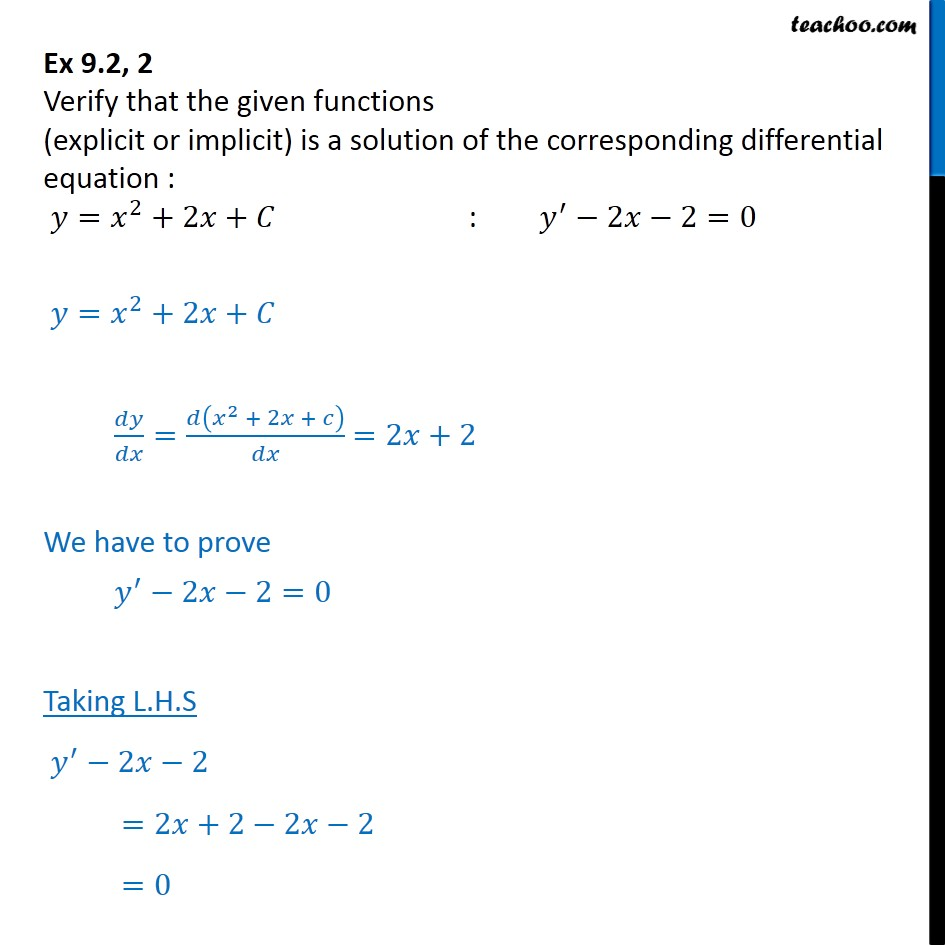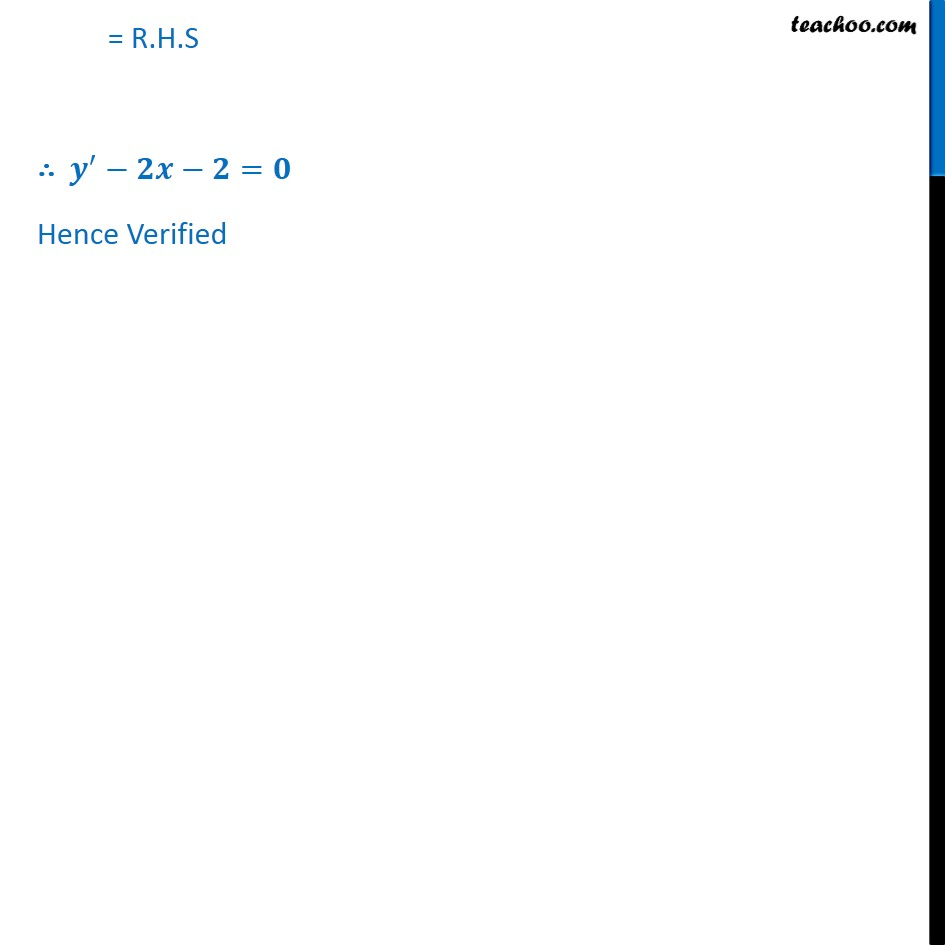Ex 9.2

Chapter 9 Class 12 Differential Equations
Serial order wiseLearn in your speed, with individual attention - Teachoo Maths 1-on-1 Class

### Transcript

Ex 9.2, 2 Verify that the given functions (explicit or implicit) is a solution of the corresponding differential equation : = 2 +2 + : 2 2=0 = 2 +2 + = 2 + 2 + =2 +2 We have to prove 2 2=0 Taking L.H.S 2 2 =2 +2 2 2 =0 = R.H.S = Hence Verified